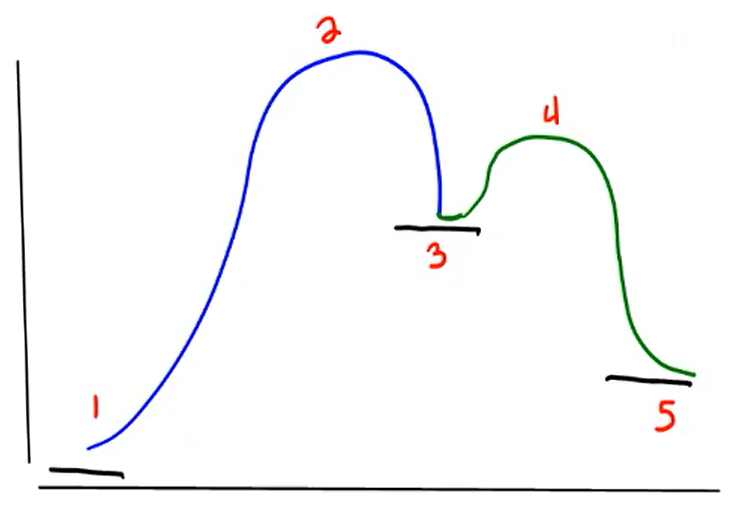Problem: Classify states 2–4 in the potential energy diagram.A. 2, 3, and 4 are transition statesB. 2, 3, and 4 are reactive intermediatesC. 2 and 4 are transition states; 3 is a reactive intermediateD. 2 and 4 are reactive intermediates; 3 is a transition state

FREE Expert Solution
81% (440 ratings)
Problem Details

Classify states 2–4 in the potential energy diagram.A. 2, 3, and 4 are transition states

B. 2, 3, and 4 are reactive intermediates

C. 2 and 4 are transition states; 3 is a reactive intermediate

D. 2 and 4 are reactive intermediates; 3 is a transition state